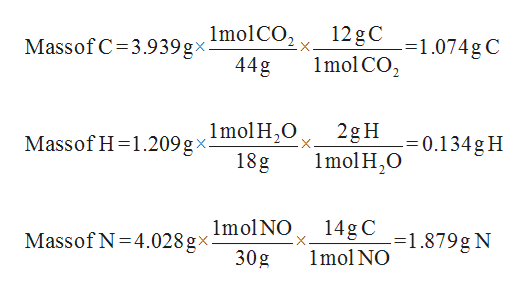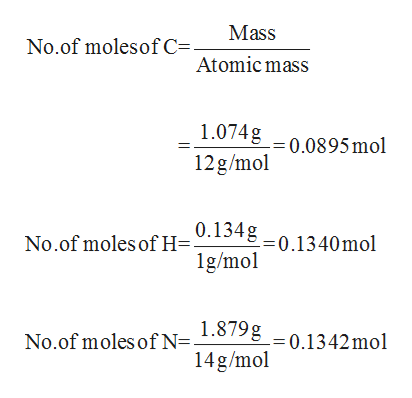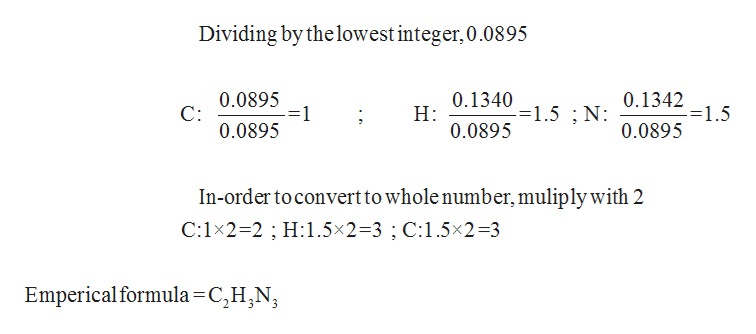# A sample of a substance (containing only C, H, and N) is burned in oxygen.3.939 g of CO2, 1.209 g of H2O and 4.028 g of NO are the sole products of combustion.What is the empirical formula of the compound? What was the mass of the initial sample burned?

Question
15 views

A sample of a substance (containing only C, H, and N) is burned in oxygen.
3.939 g of CO21.209 g of H2O and 4.028 g of NO are the sole products of combustion.
What is the empirical formula of the compound?

What was the mass of the initial sample burned?

check_circle

Step 1

Given that the mass of CO2, H2O and NO is 3.939 g , 1.209 g and 4.028 g respectively.

The mass of Carbon, Hydrogen and Nitrogen can be calculated using the simple mole concept.help_outlineImage Transcriptionclose12 gC1.074gC 1mol CO2 MassofC 3.939 gx molCO, 44g Massof H 1.209 g xmol H2O 18g 2g H 1molH2O =0.134g H 14gC1.879gN Imol NO Massof N 4.028gx. 1mol NO 30g fullscreen
Step 2

Number of moles of Carbon, Hydrogen and Oxygen can be calculated by the equation given below:help_outlineImage TranscriptioncloseMass No.of molesof C=. Atomic mass 1.074g0,0895 mol 12g/mol No.of moles of H=948-0.1340mol 1g/mo No.of moles of N1.1g0.1342mol 14g/mol fullscreen
Step 3

In-order to get the empirical formula, first the number of moles has to be divided with the lowest...help_outlineImage TranscriptioncloseDividing by the lowest integer,0.0895 0.1340 Н: 0.0895 0.0895 С: 0.0895 0.1342 -=1.5 -=1.5 ; N: =1 0.0895 In-order to convert to whole number, muliply with 2 C:1x2=2 H:1.5x2-3 ; C:1.5x2 3 Emperical formula =C,H,N fullscreen

### Want to see the full answer?

See Solution

#### Want to see this answer and more?

Solutions are written by subject experts who are available 24/7. Questions are typically answered within 1 hour.*

See Solution
*Response times may vary by subject and question.
Tagged in

### General Chemistry